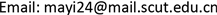Boiti-Leon-Pempinelle方程，哈密顿函数，分支理论，异宿轨, Boiti-Leon-Pempinelle Equation Hamiltonian Function Bifurcation Method Heteroclinic Orbits
Boiti-Leon-Pempinelle方程新的行波解<sup> </sup>1. 引言

{ u y t = ( u 2 ) x y − u x x y + 2 v x x x , v t = v x x + 2 u v x , (1.1)

u ( x , y , t ) = u ( ξ ) ,       v ( x , y , t ) = v ( ξ ) ,       ξ = α x + β y − c t , (1.2)

− c β u = α β u 2 − α 2 β u ξ + 2 α 3 v ξ + g 1 ξ + g . (1.4)

v ξ = 1 2 α 3 ( − c β u − α β u 2 + α 2 β u ξ − g ) . (1.5)

u = − 1 2 α ( c + α 2 v ξ ξ v ξ ) . (1.6)

v ξ ξ = 1 2 α 3 ( − c β u ξ − 2 α β u u ξ + α 2 β u ξ ξ ) . (1.7)

α 4 β u ξ ξ − α 2 g 1 − ( 2 α u + c ) ( c β u + α β u 2 + g ) = 0. (1.8)

{ d u d ξ = y , d y d ξ = 2 α 2 u 3 + 3 c α 3 u 2 + 1 α 4 β ( c 2 β + 2 α g ) u + c g α 4 β , (1.9)

H ( u , y ) = 1 2 y 2 − 1 2 α 2 u 4 − c α 3 u 3 − 1 2 α 4 β ( c 2 β + 2 α g ) u 2 − c g α 4 β u = h , (1.10)

1) 本文在函数 u ( x , t ) 之上建立了系统(1.1)一个新的哈密顿函数。

2) 基于定性理论和哈密顿函数，我们证明了该系统异宿轨的一个有趣现象，即异宿轨的存在与积分常数g无关。

3) 应用动力系统的分支理论，我们得到了系统(1.1)的平滑和非平滑行波解的精确表达式。

2. 主要结论

Δ = ( c a ) 2 − 2 g α β − u 1 2 ,     d 1 = 2 u 1 + c a < 0 ,     c 1 = Δ − u 1 − c 2 a ,

a 1 = c 1 u 1 ,     b 1 = − d 1 ( Δ + c 2 α ) ,     k 1 2 = − 2 d 1 Δ c 1 2 ,

ω 1 = | c 1 | 2 α ,     a 2 = − c 1 ( c a + u 1 ) ,     b 2 = d 1 ( Δ − c 2 α ) ,

u + = 1 2 ( − c a + c 2 a 2 − 4 g α β ) ,     u 0 = − c 2 α ,     u − = 1 2 ( − c a − c 2 a 2 − 4 g α β ) .

1) 三个周期波解

u 1 ( x , y , t ) = a 1 + b 1 sn 2 ( ω 1 ξ , k 1 ) c 1 + d 1 sn 2 ( ω 1 ξ , k 1 ) , (2.1)

u 2 ( x , y , t ) = c 1 u 2 − 2 Δ u 1 sn 2 ( ω 1 ξ , k 1 ) c 1 − 2 Δ sn 2 ( ω 1 ξ , k 1 ) , (2.2)

u 3 ( x , y , t ) = a 2 + b 2 sn 2 ( ω 1 ξ , k 1 ) c 1 + d 1 sn 2 ( ω 1 ξ , k 1 ) . (2.3)

2) 三个扭结波解

u 4 ( x , y , t ) = u − + u − − u + exp ( ± c α 2 ξ ) − 1 , (2.4)

u 5 ( x , y , t ) = u + + u + − u − exp ( ± ξ α c 2 a 2 − 4 g α β ) − 1 , (2.5)

u 6 ( x , y , t ) = u + + u − − u + exp ( ξ α c 2 a 2 − 4 g α β ) + 1 . (2.6)

3. 理论推导

f ( u ) = 2 α 2 u 3 + 3 c α 3 u 2 + 1 α 4 β ( c 2 β + 2 α g ) u + c g α 4 β . (3.1)

f ( u ) 有三个零点 u + , u 0 , u − (如图1)，由下式给出

u + = 1 2 ( − c a + c 2 a 2 − 4 g α β ) ,     u 0 = − c 2 α ,     u − = 1 2 ( − c a − c 2 a 2 − 4 g α β ) . (3.2)

y = ± 1 α ( u 1 − u ) ( u 2 − u ) ( u 3 − u ) ( u 4 − u ) ,     u ≤ u 1 , (3.3)

± ∫ u u 1 1 ( u 1 − s ) ( u 2 − s ) ( u 3 − s ) ( u 4 − s ) d s = 1 α ∫ 0 ξ d s , (3.4)

4. 结论